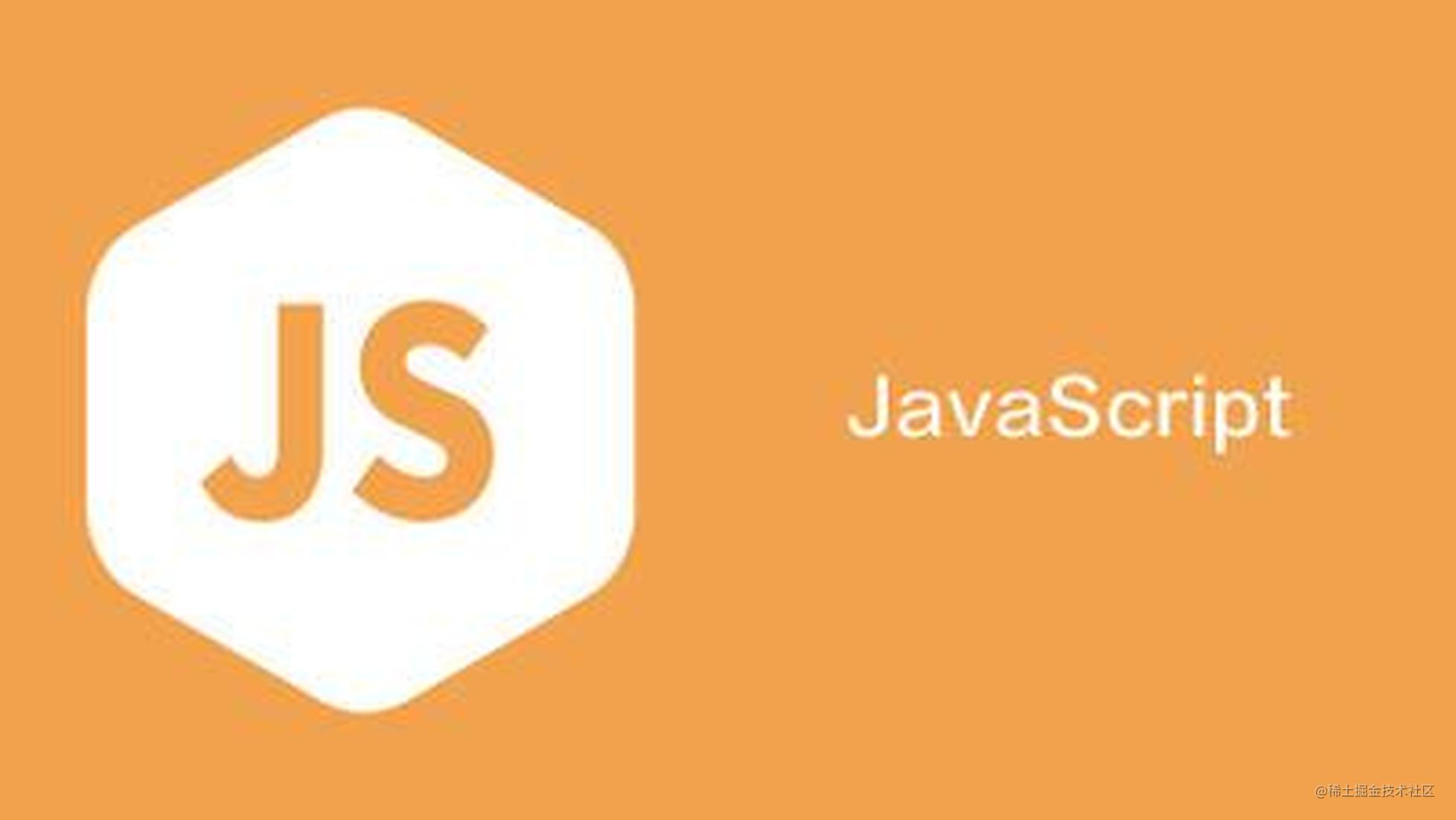JS箭头函数之：为何用？怎么用？何时用？• 更简短的函数；
• 更直观的作用域和this的绑定(不绑定this) 因为这些优点，箭头函数比起其他形式的函数声明更受欢迎。比如，受欢迎的airbnb eslint configuration库会强制使用JavaScript箭头函数创建匿名函数。 然而，箭头函数有优点，也有一些“缺点”。这就需要在使用的时候做一些权衡。下面就从为何用、怎么用、何时用，这个三部分做一些总结。

为何用？

更简短的函数

var elements = ['h1', 'div', 'span', 'section'];

elements.map(function(el) {
return el.length; // 返回数组: [2, 3, 4, 7]
});

// 从上面的普通函数可以改写为如下的箭头函数
elements.map((el) => {
return el.length; // [2, 3, 4, 7]
});

// 当箭头函数只有一个参数时，可以省略参数的圆括号
elements.map(el => {
return el.length; // [2, 3, 4, 7]
});

// 当箭头函数体只有一个`return`语句时，可以省略`return`关键字和方法体的花括号
elements.map(el => el.length); // [2, 3, 4, 7]

// 在这个例子中，因为我们只需要`length`属性，所以可以使用参数结构
// 需要注意的是字符串`"length"`是我们想要获得的属性名称，而`elLength`则只是个变量名，可以替换成任意合法的变量名
elements.map(({ "length": elLength }) => elLength); // [2, 3, 4, 7]

不绑定this

const obj = {
name: 'test object',
createAnonFunction: function() {
return function() {
console.log(this.name);
return this;
}
},
createArrowFunction: function() {
return () => {
console.log(this.name);
return this;
}
}
}

const anon = obj.createAnonFunction();
anon(); // undefined
anon() === window // true

const arrow = obj.createArrowFunction();
arrow(); // 'test object'
arrow() === obj // true

通过call或者apply调用

base: 1,
var f = v => v + this.base;
return f(a);
},
var f = v => v + this.base;
var b = {
base: 2
};
return f.call(b, a)
}
}

不绑定arguments

function foo(n) {
var f = () => arguments + n; // 隐式绑定 foo 函数的arguments对象，arguments是 n
return f();
}

foo(1); // 2

function foo(arg) {
var f = (...agrs) => args;
return f(arg);
}
foo(1); // 1

function foo(arg1, arg2) {
var f = (...args) => args;
return f(arg1, arg2);
}
foo(1, 2); // 2

怎么用?

优化代码

const words = ['hello', 'WORLD', 'Whatever'];
const downcasedWords = words.map(word => word.toLowerCase());

const names = objects.map(object => object.name);

this.examples.forEach(example => {
this.runExample(example);
});

Promise和Promise链

this.doSomethingAsync().then((result) => {
this.storeResult(result);
});

对象转换

export default {
computed: {
...mapState([
'results',
'users'
])
}
}

何时用？(不推荐使用场景)

使用new操作符

var Foo = () => {};
var foo = new Foo(); // TypeError: Foo is not a constructor

使用prototype属性

var Foo = () => {};
console.log(Foo.prototype); // undefined

使用yield关键字

yield 关键字通常不能在箭头函数中使用（除非是嵌套在允许使用的函数内）。因此，箭头函数不能用作生成器。

深层调用

{anonymous}(){anonymous}(){anonymous}(){anonymous}(){anonymous}() //anonymous

常见错误

返回对象字面量

var func = () => { foo: 1 };
func(); // undefined

var func = () => { foo: function() {} };
// SyntaxError: function statement requires a name

var func = () => ({foo: 1});

换行

var func = ()
=> 1;
// SyntaxError: expected expression, got '=>'

解析顺序

let callback;

callback = callback || function() {}; // ok

callback = callback || () => {};
// SyntaxError: invalid arrow-function arguments

callback = callback || (() => {});    // ok

更多示例

使用三元运算符

var foo = a => a > 15 ? 15 : a;

foo(10); // 10
foo(16); // 15

闭包

// 标准的闭包函数
var i = 0;
return function() {
return (++i);
}
}

// 箭头函数体的闭包(i = 0是默认参数)
var Add = (i = 0) => { return (() => (++i)) };

// 因为仅有一个返回，return及括号也可以省略
var Add = (i = 0) => () => (++i);

箭头函数递归

var fact = (x) => ( x == 0 ?  1 : x*fact(x-1) );
fact(5);       // 120